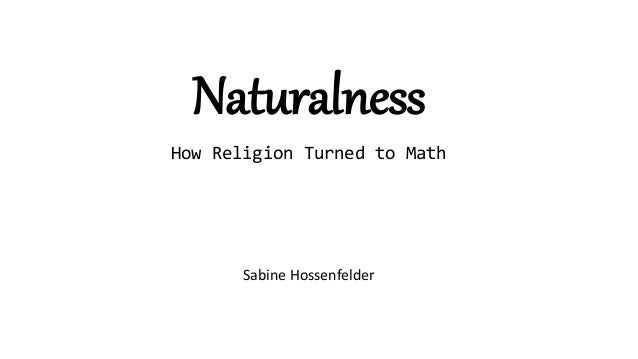Successfully reported this slideshow.Upcoming SlideShare
×

# Naturalness - How Religion turned to Math

673 views

Published on

I discuss the use and abuse of arguments from naturalness and finetuning in the foundations of physics.

Published in: Science
• Full Name
Comment goes here.

Are you sure you want to Yes No• Be the first to like this

### Naturalness - How Religion turned to Math

1. 1. Sabine Hossenfelder Naturalness How Religion Turned to Math
2. 2. The Problemwith Heliocentrism • If the Earth moves, the stars should move. • The parallax couldn’t be observed. • Astronomers concluded the stars are much farther away than the planets. • They also, mistakenly, thought the stars would have to be gigantically large. • This seemed not natural.
3. 3. Origins “It is necessary to preserve in these matters some decent proportion, lest things reach out to infinity and the just symmetry of creatures and visible things concerning size and distance be abandoned: it is necessary to preserve this symmetry because God, the author of the universe, loves appropriate order, not confusion and disorder.” ~ Tycho Brahe
4. 4. Dirac and the Large Numbers “Any two of the very large dimensionless numbers occurring in Nature are connected by a simple mathematical relation, in which the coefficients are of the order of magnitude unity.” ~ Paul Dirac 𝑇0 𝑒2/(𝑚e 𝑐2) ≈ 𝑒2 𝐺 𝑚p 𝑚e age of universe time it takes light to cross an atom gravitational force between electron and proton em force between electron and proton
5. 5. Naturalness (general) • Dimensionless numbers shouldn’t be much larger or smaller than one. • Unnatural numbers are “finetuned.” • They require an explanation. • Rationale: A finetuned number is unlikely.
6. 6. Naturalness (technical) • Applicable to quantum field theory • A dimensionless number much smaller than one must be protected by a symmetry or its smallness must be explained by some underlying physics. • Naturalness requires that numbers are not highly sensitive to the (presumed more fundamental) theory in the UV • Various quantitative measures exist.
7. 7. Theory Space
8. 8. Naturalness Works! • Smallness of electron mass: new physics no later than ~ 70 MeV → positron (.5 MeV) • Smallness of difference between mass of neutral and charged pions: new physics no later than ~ 850 MeV → r-meson (770 MeV) • Absence of flavor changing neutral currents: new physics no later than 2 GeV → charm quark (1.3 GeV)
9. 9. Naturalness Doesn’t Work • Cosmological constant: 30 orders of magnitude smaller than what the Planck-scale cutoff suggests. • Where’s the axion? To solve the strong CP problem, protect the smallness of q parameter by the Pecci-Quinn U(1) symmetry. The particle associated to the field hasn’t been found. • Higgs mass: Is 15 orders of magnitude smaller than what the Planck- scale/GUT cutoff suggests. This means new physics no later than a TeV. • The WIMP miracle: What about it?
10. 10. Naturalness at the LHC • The Higgs is the only known (fundamental) scalar. Its mass receives a contribution from quantum effects. • This contribution is assumed to be of the order of the cutoff scale, much to large. • This could be cancelled by an almost but not exactly equal bare mass by assumption. But this would be finetuned. • Supersymmetry cancels the quantum contribution. When broken, it leaves a contribution at the susy-breaking scale. • That susy wasn’t found at the LHC so far means the SM isn’t natural.
11. 11. Naturalness Has No Rationale • All axioms are infinitely unlikely anyway. What’s the big deal with a constant? • “Unlikely” requires a probability distribution. Where does this distribution come from? It requires a choice. • Technical naturalness: If there’s a TOE, what distribution are we even talking about? And why fix parameters at the Planck scale and not anywhere else? • Naturalness is an aesthetic criterion, not a mathematical one. • It’s a hypothesis with a questionable track record.
12. 12. Inflation Troubles Inflation was meant to solve three finetuning problems: 1. The homogeneity/horizon problem. Relies on the presumption of initial state which itself requires knowledge about what’s likely in a theory we don’t know. Also, it doesn’t solve it. 2. The monopole problem. Which monopole problem? 3. The flatness problem. The cure’s worse than the disease.
13. 13. Sometimes it works, sometimes it doesn’t • We don’t understand coincidence • We don’t understand exactly under which circumstances naturalness is useful. • Believing finetuning is a problem worth of study didn’t lead us anywhere.
14. 14. Consequences For Experiment • Where are the axions? • Where are the WIMPs? • Where are the Superpartners? • Where are all the funky fields supposed to explain the cosmological constant? Is there any simpler axiom than a constant parameter?
15. 15. Consequences For Theory • Wasted time. • No progress. • And multiverses. Any theory which describes our observations is necessarily infinitely finetuned. Not even the mathematical universe changes anything about this.
16. 16. Beauty in Theoretical Physics (as observed) • Simplicity: Reduce number of axioms/unify principles. Problem: Can only be used to distinguish between competing theories. • Naturalness: No large or small numbers. Problem: Ill-defined. • Elegance: Surprising consequences from sparsity. Rigidity. Problem: Depends on expectation, education, and flexibility. • Beauty: A combination of simplicity, naturalness, and elegance.
17. 17. Conclusions • Naturalness is not a mathematical criterion. • We need to rethink what is and isn’t a problem. • If intuition fails, more rigor is required, not less.